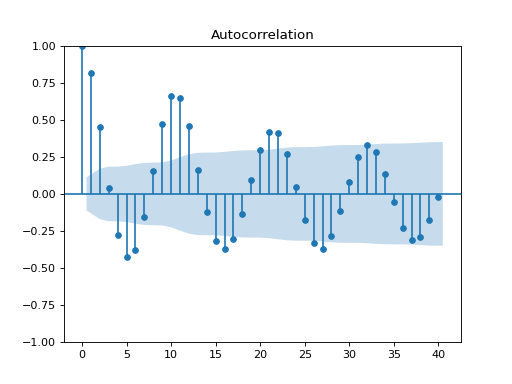# statsmodels.graphics.tsaplots.plot_acf¶

statsmodels.graphics.tsaplots.plot_acf(x, ax=None, lags=None, alpha=0.05, use_vlines=True, unbiased=False, fft=False, title='Autocorrelation', zero=True, vlines_kwargs=None, **kwargs)[source]

Plot the autocorrelation function

Plots lags on the horizontal and the correlations on vertical axis.

Parameters
• x (array_like) – Array of time-series values

• ax (Matplotlib AxesSubplot instance, optional) – If given, this subplot is used to plot in instead of a new figure being created.

• lags (int or array_like, optional) – int or Array of lag values, used on horizontal axis. Uses np.arange(lags) when lags is an int. If not provided, lags=np.arange(len(corr)) is used.

• alpha (scalar, optional) – If a number is given, the confidence intervals for the given level are returned. For instance if alpha=.05, 95 % confidence intervals are returned where the standard deviation is computed according to Bartlett’s formula. If None, no confidence intervals are plotted.

• use_vlines (bool, optional) – If True, vertical lines and markers are plotted. If False, only markers are plotted. The default marker is ‘o’; it can be overridden with a marker kwarg.

• unbiased (bool) – If True, then denominators for autocovariance are n-k, otherwise n

• fft (bool, optional) – If True, computes the ACF via FFT.

• title (str, optional) – Title to place on plot. Default is ‘Autocorrelation’

• zero (bool, optional) – Flag indicating whether to include the 0-lag autocorrelation. Default is True.

• vlines_kwargs (dict, optional) – Optional dictionary of keyword arguments that are passed to vlines.

• **kwargs (kwargs, optional) – Optional keyword arguments that are directly passed on to the Matplotlib plot and axhline functions.

Returns

fig – If ax is None, the created figure. Otherwise the figure to which ax is connected.

Return type

Matplotlib figure instance

See also

matplotlib.pyplot.xcorr, matplotlib.pyplot.acorr, mpl_examples

Notes

Adapted from matplotlib’s xcorr.

Data are plotted as plot(lags, corr, **kwargs)

kwargs is used to pass matplotlib optional arguments to both the line tracing the autocorrelations and for the horizontal line at 0. These options must be valid for a Line2D object.

vlines_kwargs is used to pass additional optional arguments to the vertical lines connecting each autocorrelation to the axis. These options must be valid for a LineCollection object.

Examples

>>> import pandas as pd
>>> import matplotlib.pyplot as plt
>>> import statsmodels.api as sm

>>> dta = sm.datasets.sunspots.load_pandas().data
>>> dta.index = pd.Index(sm.tsa.datetools.dates_from_range('1700', '2008'))
>>> del dta["YEAR"]
>>> sm.graphics.tsa.plot_acf(dta.values.squeeze(), lags=40)
>>> plt.show()